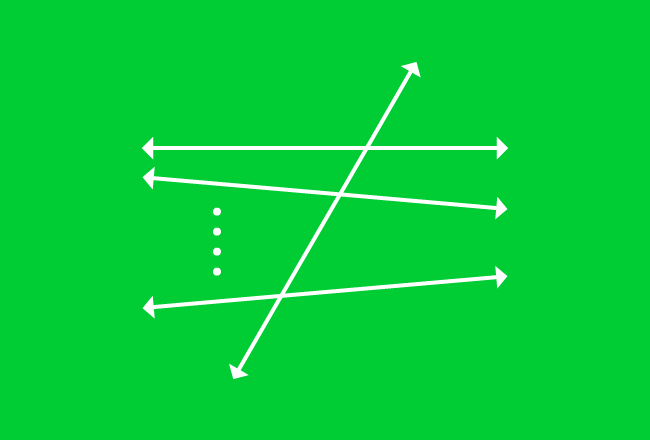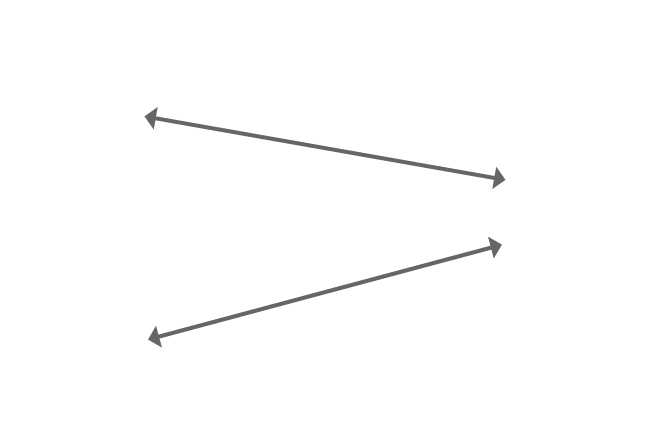# Transversal of Nonparallel LinesA straight line that intersects either two or more nonparallel lines is called a transversal line of nonparallel lines.

In geometric system, a straight line often intersects two or more non-parallel lines in some cases. It actually cuts the straight line at distinct points on a plane. It is called a transversal and also called as transversal line.

The picture represents the formation of nonparallel lines cut by a transversal geometrically.

## Example

Two nonparallel lines cut by their transversal is another most useful geometrical concept.$\overleftrightarrow{AB}$ and $\overleftrightarrow{CD}$ are two nonparallel lines. $\overleftrightarrow{XY}$ is a straight line but it cuts both non-parallel lines $\overleftrightarrow{AB}$ and $\overleftrightarrow{CD}$ at different intersecting points $P$ and $Q$ respectively.

Therefore, the straight line $\overleftrightarrow{XY}$ is called as a transversal line or simply transversal of nonparallel lines $\overleftrightarrow{AB}$ and $\overleftrightarrow{CD}$. It is also called as nonparallel lines $\overleftrightarrow{AB}$ and $\overleftrightarrow{CD}$ cut by their transversal line $\overleftrightarrow{XY}$.

Latest Math Topics
Jun 26, 2023
Jun 23, 2023

Latest Math Problems
Jul 01, 2023
Jun 25, 2023
###### Math Questions

The math problems with solutions to learn how to solve a problem.

Learn solutions

Practice now

###### Math Videos

The math videos tutorials with visual graphics to learn every concept.

Watch now

###### Subscribe us

Get the latest math updates from the Math Doubts by subscribing us.